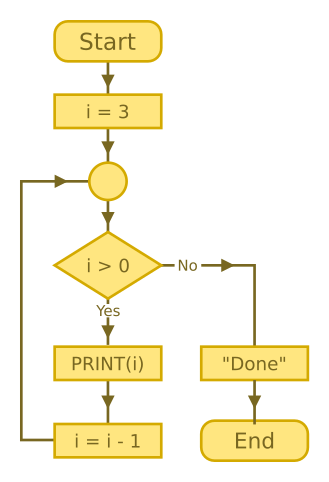# While loops

Martin McBride, 2017-02-03
Tags none
Categories none

In programming, iteration simply means repeating the same block of code multiple times. A common way to that is a loop.

There are 2 main types of loop:

• while loops - loop while ever a particular condition is true.
• for loops - typically count a fixed number of times.

## While loops

Here is a simple while loop:

INT i = 3
WHILE i > 0
PRINT(i)
i -= 1
ENDWHILE
PRINT("Done")


The meaning of this code can easily be expressed in plain English:

Start with i equal to 3. While ever i is greater than 0, we print the value of i, then reduce the value of i by 1. Finally, print Done.

You can probably work out what this program will print:

3
2
1
Done


Here is a flowchart of the while loop code:Here is a video that steps through the code, line by line:

## Do-while loops

A do-while loop is similar to a while loop, except that the condition is at the end. This means that the code inside the loop will always run at least once. It is useful if you need to do something before you check the loop condition.

In this example, the program asks the user a simple question, and then loops until they enter the correct answer. It makes so sense to check the answer before you have asked the question, so we use a do-while loop.

INT answer
DO
PRINT("What is 2 + 2?")"
PRINT("Correct")


We declare our variable answer, then enter the loop. First we ask a question "What is 2 + 2?", then we allow the user to enter an answer.

Then we check the answer value. The operator != means not equal. This means that if answer is not equal to 4, the condition is true, so the code jumps back to the start of the loop. It asks the question again, inputs the answer and checks the value ... and repeats until the user inputs 4. Here is what the interaction looks like:

What is 2 + 2?
> 3
What is 2 + 2?
> 7
What is 2 + 2?
> 4
Correct


## Repeat-until loops

A repeat-until loop is very similar to a do-while loop. The difference is:

• A do-while loop continues looping while ever the condition is true.
• A repeat-until loop continues looping while ever the condition is false.

So we can easily convert of do-while into a repeat-until simple by changing the condition from != (not equal) to == (equal):

INT answer
REPEAT
PRINT("What is 2 + 2?")"
PRINT("Correct")


## Which sort of loop should I use?

The first thing to remember is that you can always use a while loop. For example, this do-while loop:

DO
dostuff()
WHILE condition


is exactly the same as this while loop:

dostuff()
WHILE condition
dostuff()
ENDWHILE


So do-while is really an optional extra. Use it if you think it makes your code a bit tidier.

{{% purple-note %}} Python doesn't even have a do-while or repeat-until loop. It only has a while loop. {{% /purple-note %}}